A supply plane needs to drop a package of food to scientists working on a glacier in Greenland. The plane flies 110 m above the glacier at a

Question

A supply plane needs to drop a package of food to scientists working on a glacier in Greenland. The plane flies 110 m above the glacier at a speed of 150 m/s . You may want to review (Page) . For help with math skills, you may want to review:

in progress 0
5 months 2021-09-03T06:18:37+00:00 1 Answers 6 views 0

The distance is 709.5 m.

Explanation:

Given that,

Speed = 150 m/s

Distance = 110 m

Suppose, How far short of the target should it drop the package?

We need to calculate the time

Using equation of motion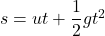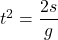Where, g = acceleration due to gravity

t = time

Put the value into the formula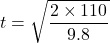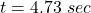We need to calculate the distance

Using formula of distance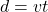Put the value into the formula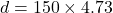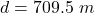Hence, The distance is 709.5 m.